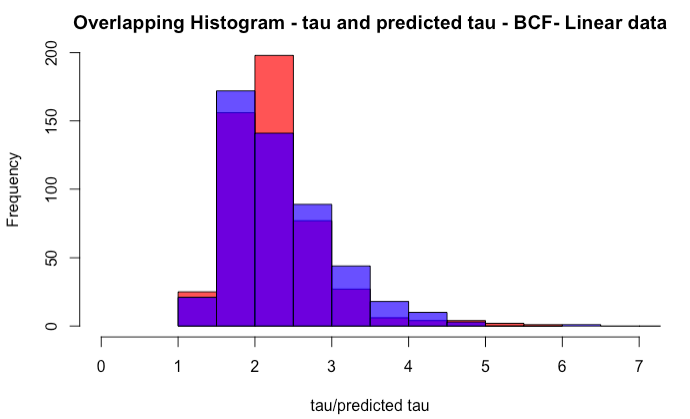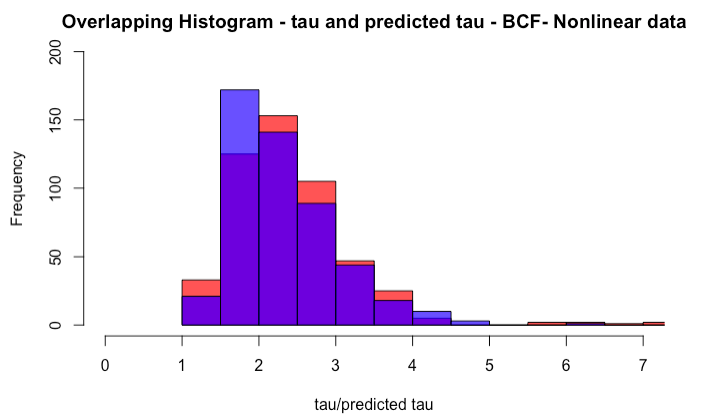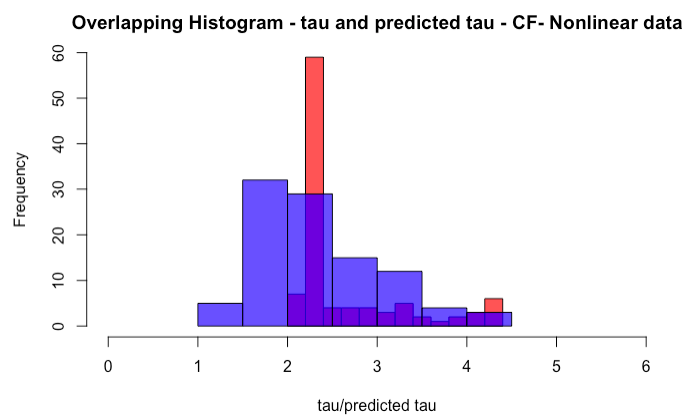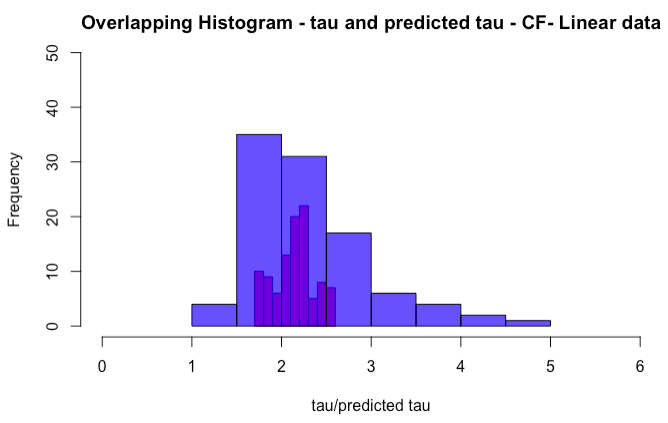# Heterogeneous treatment effects : linear and non-linear data

Comparison between linear regression, causal forest, and Bayesian causal forest models for causal inference in the presence of heterogeneous treatment effects : linear and non-linear data

Authors: Devin Khosla and Jui Nerurkar

Motivation:

In research, we often want to make causal claims about the treatment effect on a given population. However, “historically most datasets have been too small to meaningfully explore heterogeneity of treatment effects beyond dividing the sample into a few subgroups” (Wager & Athey, 2015). Furthermore, there is a concern that when looking for heterogeneous treatment effects, subsetting data multiple times may lead to spurious effects. Using simulated data, we will compare how well three different methods estimate the treatment effect given heterogeneous treatment effects in our data. The three methods we chose to examine are linear regression, causal forests and Bayesian causal forests.

For our analysis, the estimand of interest is the average treatment effect (ATE). This is because while our sample was assigned treatment or not, they may all choose to receive the treatment outside of the study. Furthermore, for a drug that could improve the health of someone, we are interested to see how it would effect all people not just a smaller sample of the treated for example.

As discussed in Wager & Athey, nearest neighbour matching, kernel methods and series estimation do perform well in estimating heterogeneous treatment effects but as the number of covariates increases, these methods quickly breakdown. Wager & Athey (2017), develop a causal forest method which they use to estimate treatment effects. The second method we are interested in using is the Bayesian causal forest approach developed by Hahn, Murray and Carvalho. Finally as a baseline, we will also estimate the treatment effect using linear regression. While we do not expect this to perform well, it will be helpful as a comparison to both forest techniques.

Assumptions:

When simulating the data we wanted to use, we had to define some assumption for our data generating process. This next section describes these assumptions. Let X denote an n × p matrix of features, Z denote a binary treatment and Y be a vector of quantitative responses, where Y ∈ Rⁿ,  n is the number of observations in our dataset, and p is the number of predictors. Further, let the lowercase letter xᵢ denote the observed  feature set of length p, zᵢ denote the treatment assigned and yᵢ be the observed outcome for a given individual, i.  Following the Neyman-Rubin potential outcomes model, each individual i has potential outcomes Yᵢ(1) and Yᵢ(0), which would be observed under Z = 1 and Z = 0, respectively. The fundamental problem of causal inference is that only one of these potential outcomes can be observed, Yᵢ = Yᵢ(1)Zᵢ + Yᵢ(0)(1-Zᵢ). In this paper, we will consider that the observed sample consists of n independent observations (Yᵢ, Zᵢ, xᵢ), for i = 1,2,3,…n, where Y or Z, when stated without a subscript, denote column vectors of length n.

We are interested in estimating the ATE i.e the difference between the average outcome among those who received the treatment and the average outcome among those who did not receive the treatment. In randomized experiments,

ATE = E[Y| Z =1] – E[Y| Z = 0]

Throughout, we make the stable unit treatment value assumption (SUTVA) which states that there is no interference among units. The response surface, which is defined as ‘a model for the outcome (or potential outcomes) conditional on the treatment and confounding covariates’, is generated only using the covariates x, to thereby ensure that the assumption of ignorability is satisfied. The data generation process (DGP) ensures that the overlap assumption is satisfied for the treatment and control groups, which is necessary for estimating treatment effects everywhere in the covariate space. For the sake of clarity, response surface being linear implies a linear relationship between the outcome and covariates, and response surface being nonlinear implies a nonlinear relationship between them.

In addition to the above, we assume targeted selection in our synthetic DGP. In other words, selection is dependent on the function used to generate Y(0), i.e the potential outcome in the absence of treatment. This type of selection process is expected to be quite common in certain contexts, for example – where risk factors for adverse outcomes are well-understood, individuals with worse expected outcomes in the absence of treatment are more likely to be assigned to treatment.

Designs/Estimators:

We intend to evaluate the performance of linear regression, causal forest (using the default specification in the grf package (R)), and Bayesian causal forest (using the default specification in the bcf package (R)) for estimating heterogeneous treatment effects. Out of the three, causal forest (CF) and Bayesian causal forest (BCF) are non-parametric and are much more flexible as compared to linear regression.

Linear regression can be used to estimate the causal effects if the model includes all the confounding covariates and if the model is correct. In addition to the assumptions mentioned above, this method requires the following parametric assumptions – linear relationship between the deterministic component and separate predictors, independence across observations, homoskedasticity of errors, and normality of errors. If the confounding covariates are all observed, then accurate estimating depends on proper modeling and the extent to which the model is forced to extrapolate beyond the support of the data. In the cleanest scenario of random sampling and random assignment, average causal effect of the treatment can be estimated, and regression can assist in further refining the estimate. However, in an observational study, there can be systematic differences between groups of units that receive the different treatments with respect to key covariates, x, that can potentially affect the outcome. In such instances, merely satisfying ignorability might not justify using regression as the best modeling approach for estimating treatment effects. In the absence of balance and overlap, stronger reliance is placed on model specification.

With targeted selection, our data does not resemble a randomized experiment. Moreover, we have two DGP’s, one where Y is linearly related to covariates x and the other where Y has a nonlinear relation to covariates x. The treatment effect 𝛕 is heterogeneous and is a nonlinear function of the covariates. We will obtain linear regression estimates for the treatment effect by simply regressing the outcome on the treatment assignment indicator Z and the confounding covariates.

Causal forest is a non-parametric method which involves building causal trees that resemble their regression analogues i.e the classification and regression trees (CART). In a broad sense, trees or forests can be thought of as nearest neighbor methods with an adaptive neighborhood metric. The process of building trees starts by recursively splitting the feature space until it is partitioned into a set of leaves L, each of which only contains a few samples from the training set. Thereafter, given a test point x, we evaluate the prediction for the outcome by identifying the leaf L(x) containing x. In the context of causal trees, we want to think of leaves as small enough that the (Yᵢ, Zᵢ) pairs corresponding to the indices i for which i ∈ L(x) act as though they had come from a randomized experiment. Then, the estimate of treatment effect for any x ∈ L can be obtained as:

Finally, a causal forest generates an ensemble of N such trees, each of which outputs an estimate of 𝛕n (x). The forest then aggregates their predictions by averaging all those 𝛕(x) estimates. The advantage of using a causal forest over a causal tree is that it is not always evident what the ‘best’ causal tree is and hence, it is often better to generate the average of many different decent looking trees, instead of seeking to build one highly optimized tree. Moreover, this method also helps in reducing variance and smoothing sharp decision boundaries.

The Bayesian causal forest model was developed for estimating treatment effects from observational data, geared specifically towards situations with small effect sizes, heterogeneous effects, and strong confounding. This model deals with the issue of yielding biased estimates of treatment effects when fit to data with strong confounding by directly incorporating an estimate of the propensity function in the specification of the response model. BCF consists of flexible sum-of-regression-trees to model a response variable as a function of a binary treatment indicator and a vector of control variables. This model uses a prior for the response surface that depends explicitly on the estimates of the propensity score as a 1- dimensional transformation of the covariates. Though incorporating this transformation is not absolutely necessary, including it substantially improves the treatment effect estimation in the presence of moderate to strong confounding , especially when the confounding is driven by targeted selection.

Further, the regression in this model is presented as a sum of two functions: the first models the prognostic impact of the control variables, i.e the component of the conditional mean of the response that is unrelated to the treatment effect, while the second represents the treatment effect directly, which itself is assumed to be a nonlinear function of the observed attributes, possibly capturing heterogeneous treatment effects. BCF performs dramatically better in cases involving strong confounding, targeted selection, and relatively weak treatment effects, which is commonly seen in applied settings.

Bayesian additive regression tree (BART) is designed to estimate a model for the outcome Y, such that, Yᵢ = 𝑓(xᵢ, Zᵢ) + ϵᵢ, where ϵᵢ ~ Normal (0, 𝛔²), and E(Yᵢ | xᵢ, Zᵢ = zᵢ) = f (xᵢ, zᵢ). Previous work (Hill, 2011) advocated using a BART prior for f (xᵢ, zᵢ) directly. However, in this model the response surface is alternately expressed as,

where the functions 𝝁 and 𝛕 are given independent BART priors and (xi) is an estimate of the propensity score (xᵢ) = Pr(Zᵢ = 1|xᵢ).

Aside from the parametric and non-parametric methods distinction between linear regression and the other two methods, the methodologies used for estimating the treatment effects are almost quite different in these two groups of methods. One of the main differences between causal forest and Bayesian causal forest is that causal forest is based on the random forest algorithms while the latter is based on BART. Moreover, prior research has shown that the presence of highly correlated covariates has an adverse effect on the predictions made by the random forest model. However, this doesn’t seem to affect the BART model.

In our simulations, we have two DGPs, linear and nonlinear. The treatment effect is heterogeneous and a nonlinear function of the covariates. Selection into treatment group is targeted. However, we have ensured that there is sufficient overlap for both the response surfaces. Given the structural and parametric assumptions of all our models and our DGPs, we believe linear regression and causal forest would face several challenges in accurately predicting treatment effects.

Simulation:

For our simulation, we chose to run 200 iterations. Due to the computational complexity of our methods, running more than 200 iterations would be too time intensive. We are interested in looking at a simulated dataset using a medical context. Our outcome variable is an overall health rating. Our dataset includes 1000 people who had malignant tumors, received treatment and we measured variables some time after their treatment. The data we have generated includes each individual’s weight, age, the size of the tumor, their systolic blood pressure, gender and whether they are in remission or not. Using this data, we will attempt to estimate the average treatment effect for the patients in our study. Specifically our research question is, what difference in the health measure would we expect to observe if all the patients in our study received the treatment as opposed to none of them receiving the treatment?

We create this data by drawing from a multivariate normal distribution. This is because in the real world, there is often a correlation between the predictors which we wanted to emulate in our data. By doing this, we can create these correlations in the data. After creating the data, we create a vector of Betas and we use the betas to generate the outcome variable. We first use matrix multiplication to create data using a linear process and the betas we have chosen. We also create a second dataset where the outcome variable is generated using a non-linear method.

To summarize, in our simulations we need the following elements for our data generating processes:

1. A matrix X using six variables; first four are strongly correlated continuous, drawn from a multivariate normal distributions, the fifth is a dichotomous variable randomly drawn from the Bernoulli distribution and independent of the other variables, and the sixth is a dichotomous variable which is also randomly drawn from a Bernoulli distribution but is dependent on the third variable.
2. We use the following formula to generate the 𝛕:
1. The linear potential outcome variable, Y0 is generated by multiplying matrix X with slopes 𝛃 and adding errors, ϵ ~ Normal (0,1).
2. The potential outcome variable, Y1 in both DGPs is generated using the same equations that generate Y0 under that particular setting and adding 𝛕 to it.
3. Below are the two methods used to generate the outcome variables:

In both cases, the epsilon is an error that is drawn from a standard normal distribution.

When looking at the three methods, we want to see how each performs using both datasets and see if as hypothesized, the forest methods do much better when the data is non-linear. In both cases we do not have a random treatment assignment but a targeted assignment. This is because we would want individuals who are considered more likely to have adverse outcomes in the absence of treatment  to be assigned treatment. The probability of treatment assignment is therefore created using their weight, age, tumor size and whether they relapsed. Using this data, we created train and test sets which we will use for our linear regression and causal forests. We chose an 80-20 split for this.

Now that we have the data, we start modeling. The first models we create are linear regressions on the training data for both the linear and non-linear data. Using these models we predict the outcome variable on the respective test sets and then generate our estimates of the treatment effects. Next we use causal forests on the training data and predict the outcomes on the test set. Using this, we calculate the treatment effects for this data. Using the same approach we are able to calculate the treatment effect from the Bayesian causal forest approach. Finally we also decided to re-run this same simulation with a sample size of 500. We did this as we were interested to see what how this might change the model diagnostics.

Simulation results:

To evaluate the performance of the three methods, we consider how each method does at estimating the (sample) average treatment effect (ATE) using the normalized root mean squared error (RMSE) and bias.

The summary of the results is as follows:

• BCF has the least RMSE under both the response surfaces;
• Causal forest exhibits subpar performance;
• The RMSE for linear regression under both the response surfaces is almost equal, but it is smaller than the RMSE for causal forest in both instances;

Table 1: Simulation study results under both the DGP settings. Root mean squared error (RMSE) and bias are reported for all three methods.

BCF was developed to deal with heterogeneous and nonlinear treatment effects, and strong confounding. The simulated data under the first response surface had a linear relationship between the outcome variable and the covariates, however the treatment effect was heterogeneous and nonlinear. In this situation, linear regression in unfairly disadvantaged and hence, fails to be a strong competitor for BCF.

Causal forest fails to perform in both the settings and surprisingly, the RMSE for linear regression is smaller than that for causal forest even when the response surface is nonlinear. Moreover, causal forest’s performance further deteriorates under the nonlinear response surface.

When n = 500, the bias for BCF decreases slightly under the nonlinear DGP as compared to the linear DGP. However, when n  is increased to 1000, bias for the BCF model under both the settings is almost the same. Linear regression has the smallest bias under the linear DGP when n= 500 and n = 1000. The bias drastically increases for causal forest under the nonlinear DGP. In general, increasing n shows a reduction in RMSE for both the forest methods.

Thus, when the data consists of:

• Strongly correlated covariates;
• Heterogeneous and nonlinear treatment effects;
• Satisfies the assumptions of ignorability and sufficient overlap

BCF gives better estimates irrespective of whether the DGP is linear or nonlinearThese histograms show the overlap between the actual treatment effects and the predicted treatment effects. The red histograms are the predicted 𝛕 values and the blue histograms are the actual 𝛕 values in all the four plots.

The BCF histograms show an impressive overlap between the actual 𝛕 and predicted 𝛕 values. On the other hand, for the linear DGP, causal forest makes predictions which cover a very small range of actual 𝛕 values. For the nonlinear DGP, causal forest predictions cover a larger range of actual 𝛕 values but the overlap is not as impressive as that of BCF.

Discussion:

When looking at data with heterogeneous treatment effects, where the predictors are highly correlated and the outcome has a nonlinear relationship with the covariates, our results seem to suggest that using Bayesian causal forests will provide the best estimates of the treatment effect. We compared the square root of the average squared treatment effects for all three models and found that the Bayesian causal forests performed the best while causal forests performed the worst. It was surprising to us that linear regression performed as well as it did as the data was generated using a nonlinear method. A possible reason for this could be that in situations with sufficient overlap, linear regression presents the best linear approximation to the true model. However upon further examination of the residuals we discovered that this may be due to the bias cancelation as the model overestimates and underestimates the treatment effects for some of our sample. An important drawback of standard linear regression is that it fails to alert the researcher when a bad decision regarding the choice of model is made.

When looking at similar data but now when the outcome is generated using a linear method, we find similar results overall. That being said, we do find that the difference between Bayesian causal forest and linear regression does narrow. Since Bayesian causal forest seems to perform the best with both linear and nonlinear data, when working with data similar to ours, we recommend using this method to researchers in the future. We also find that when the sample size increases, both the bias and RMSE for all three models decreases.

If we were to take this project a step further, we may want to look at the confidence intervals around our estimates of the treatment effects and conduct a sensitivity analysis. By doing this we would be more certain of our findings that Bayesian causal forests is the best method. Furthermore, we may want to explore what happens when our data has different levels of overlap and/or balance. In addition to the above, we might even want to explore Bayesian causal forests to obtain conditional average treatment effects (CATE), i.e the amount by which the response variable would differ between the two hypothetical treatment conditions averaged across the subpopulations defined by covariates x.